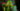# Python Easy Basics 2Welcome back to Python Easy Basics tutorial, it’s time to learn some cool functionality and break the ice with Python.

If you didn’t read the first Python Easy Basics, I recommend you to catch up on it for better understanding! CLICK MEEEEEEE

### Conditions: if and else

``````a = 1
b = 2
d = 1

if a == b:
print("a and b are the same!")
else:
print("a and b are not the same!")    #executed

if a==d:
print("a and d are the same!")
else:
print("a and d are not the same!")    #executed
``````

If statements can check a given condition. In the first example the Variables “a” and “b” get compared, if they are the same the indented code after the if statements gets executed but if they are not the same they indented code after the else statement gets executed. “a” and “b” are not the same so the indented code after the else is executed.

In the second statements “a” and “d” get compared, they are the same so the intended code after the if statement gets executed.

### Conditions: elif

``````a = "hello"

if a == "bye":
print("Goodbye!")
elif a == "hello":
print("Oh hello too!")      #executed
else:
print("What did you say?")``````

An elif Statement is the next statement that is tested. At first “a” gets compared to the string “bye”. The “hello” from “a” is not the same as “bye” so the condition of the elif gets checked which is true so the indented code after the elif gets executed.

#### List of conditions

• Equals: a == b
• Not Equals: a != b
• Less than: a < b
• Less than or equal to: a <= b
• Greater than: a > b
• Greater than or equal to: a >= b

You learned now the second part of the basics and now you’re getting better at coding! Soon there be more parts to this tutorial and you will learn loooooops!

Python Sources